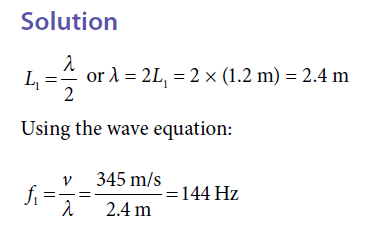# Resonant Lengths in open air column question

## Homework Statement

An organ pipe 1.2m long and open at both ends produces a note with the fundamental frequency. If the speed of sound in air is 345 m/s, what is the fundamental frequency?

## Homework Equations

Wave equation (f = v/lambda)

## The Attempt at a Solution

My textbook solves the problem like so:My question is: why do they use the full wavelength here? As I understand it, the fundamental frequency is only half a wavelength. This would be more like the second resonant length, which was not what the question asks.

#### Attachments

the fundamental frequency is only half a wavelength
First, a frequency is not a length.
Fundamental frequency means the lowest note the pipe can produce. Being open at both ends, the pipe will only contain half a wavelength. Thus, as you imply, the fundamental frequency here corresponds to a half wavelength. Hence the λ=2L.
But to find the frequency that you hear you must divide the speed of sound by the full wavelength.

•First, a frequency is not a length.
Fundamental frequency means the lowest note the pipe can produce. Being open at both ends, the pipe will only contain half a wavelength. Thus, as you imply, the fundamental frequency here corresponds to a half wavelength. Hence the λ=2L.
But to find the frequency that you hear you must divide the speed of sound by the full wavelength.

So the wavelength has to be twice the length of the pipe because the pipe is open on both sides? So the fundamental frequency corresponds to the actual length of the pipe?

How would this be solved if the pipe were closed on one end?

How would this be solved if the pipe were closed on one end?
In that case, how much of a wavelength (at fundamental frequency) would fit in the pipe?

In that case, how much of a wavelength (at fundamental frequency) would fit in the pipe?

1/4 wavelength?

Also, was the rest of the conclusion earlier correct?

1/4 wavelength?
Yes.

the fundamental frequency corresponds to the actual length of the pipe?
It might be clearer to think in terms of the "fundamental wavelength", i.e. the longest wavelength which can be produced. That is related, on the one hand, to the pipe length and its number of closed ends, and on the other to the fundamental frequency by the speed of sound in air.